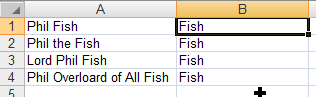### Extract a Surname from a string in ExcelThere comes a point in every Excel users workflow that they've got to work with a list of names. Usually the data you get is in the wrong format, or you have to compare two dataset of different formats. This can be frustrating but here is a quick guide of how to manipulate them.

This tutorial is based around extracting the surname / family name from a variety of different formats, if you need to get to the title or firstname then it should be easily enough to adapt the workarounds.

### Surname, Forename

Possibly the best format to get the data in, it is quick and easy to extract the surname, like everything in excel all you have to do is break the task into logical steps. Lets go with this example:

Fish, Phil the

Breaking it down, the portion of the name we want to take are all the letters before the comma. Using the `FIND` function and assuming the string is in cell A1 we'd use this formula:

`=FIND(",",A1,1)-1`

This results in the expected answer of 4. All we need to do next is use the `LEFT` formula to extract the letters:

`=LEFT(A1,4)`

We can then nest these formulas into one compact equation:

`=LEFT(A1,FIND(",",A1,1)-1)`

Et voila

Fish

### Title Forename Initial Surname

It wouldn't be so bad to extract the Surname from a Forename Surname configuration, you just repeat the above steps but instead look for a space and use the RIGHT function along with `LEN` - which tells you how many characters are in the string so you can extract the space to last letter. E.g. for

Phil Fish
`=RIGHT(A1,LEN(A1)-FIND(" ",A1))`

Again giving you

Fish

the trouble is that the data rarely comes through like this. There are several permitations which will come through including middle name/no middle name/multiple middle names and title:

Phil Fish
Phil the Fish
Lord Phil Fish
Phil Overloard of All Fish

Back to our human logic, all we really have to do here is take the letters after the last space, unfortunately this is trickier than it sounds!

To accomplish our task we have to break this down into futher steps, first off:

#### Count the number of spaces

Counting how many occurances a string has can be calculated by counting the total length of the string minus the length of the string without the text you are trying to find. Pretty cunning really, you'll have needed a bit of out of the box thinking for that one. Here's the formula to use:

`=SUM(LEN(<range>)-LEN(SUBSTITUTE(<range>,"text","")))/LEN("text")`

or in the context of our example:

Phil the Fish

this formula:

`=SUM(LEN(A1)-LEN(SUBSTITUTE(A1," ","")))/LEN(" ")`

will yield the answer of 2.

#### Find the last occurance

Now we have found how many times the space appears we have to locate the last occurance, we do this by replacing the last space with something unique which we know won't appear for this we'll use a special system charachter Null and call it using the CHAR function which retreives is using its ASCII code:

`CHAR(1)`

So as a formula we'll substitute the space in the text string and find it:

`=FIND(CHAR(1),SUBSTITUTE(A1," ",CHAR(1),2))`

This give us the position of 9,

#### Extract the surname

Next all we have to do is proceed to grab the last 9 letters of the string as described earlier and we are away:

`=RIGHT(A1,9)`

#### Condense into one formula

Finally once we've figured out all the steps we can bung all the code into one formula:

`=RIGHT(A1,LEN(A1)-FIND(CHAR(1),SUBSTITUTE(A1," ",CHAR(1),SUM(LEN(A1)-LEN(SUBSTITUTE(A1," ","")))/LEN(" "))))`

And we are away. I told you it wasn't as easy as you first imagined!